# 30 Results

View
Selected filters:
• MCCRS.Math.Content.6.EE.A.2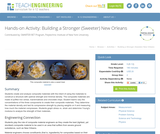Conditions of Use:
Rating

Students create and analyze composite materials with the intent of using the materials to construct a structure with optimal strength and minimal density. The composite materials are made of puffed rice cereal, marshmallows and chocolate chips. Student teams vary the concentrations of the three components to create their composite materials. They determine the material density and test its compressive strength by placing weights on it and measuring how much the material compresses. Students graph stress vs. strain and determine Young's modulus to analyze the strength of their materials.

Subject:
Architecture and Design
Engineering
Material Type:
Activity/Lab
Provider:
TeachEngineering
Provider Set:
TeachEngineering
Author:
Charisse Nelson
Sarah Wigodsky
10/14/2015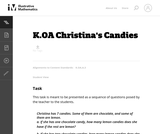Conditions of Use:
No Strings Attached
Rating

The purpose of this task is for students to find different pairs of numbers that sum to 7.

Subject:
Mathematics
Measurement and Data
Material Type:
Activity/Lab
Provider:
Illustrative Mathematics
Provider Set:
Illustrative Mathematics
Author:
Illustrative Mathematics
05/01/2012Conditions of Use:
Remix and Share
Rating

This unit is an EQuIP Exemplar for adult education (http://achieve.org/equip). Students will connect their prior, real-world knowledge to the concept of order in mathematics. They will go through a discovery process with content that will build a deep, conceptual understanding of the properties of operations to explain why we perform operations in a certain order when we see just the naked numbers.

Material Type:
Lesson Plan
Unit of Study
Provider:
EQuIP Exemplars
Author:
Connie Rivera
11/26/2014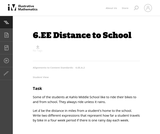Conditions of Use:
No Strings Attached
Rating

This task asks students to find equivalent expressions by visualizing a familiar activity involving distance. The given solution shows some possible equivalent expressions, but there are many variations possible.

Subject:
Mathematics
Material Type:
Activity/Lab
Provider:
Illustrative Mathematics
Provider Set:
Illustrative Mathematics
Author:
Illustrative Mathematics
05/01/2012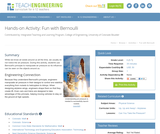Conditions of Use:
Rating

While we know air exists around us all the time, we usually do not notice the air pressure. During this activity, students use Bernoulli's principle to manipulate air pressure so its influence can be seen on the objects around us.

Subject:
Engineering
Physics
Material Type:
Activity/Lab
Provider:
TeachEngineering
Provider Set:
TeachEngineering
Author:
Alex Conner
Geoffrey Hill
Janet Yowell
Malinda Schaefer Zarske
Tom Rutkowski
10/14/2015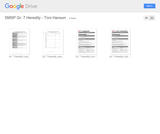Conditions of Use:
Remix and Share
Rating

Students will construct an argument based on evidence regarding their position on genetically modified organisms (GMOs). Students will conduct an inquiry investigation in Mendelian genetics using Wisconsin Fast Plants.

Subject:
Life Science
Genetics
Material Type:
Activity/Lab
Lesson Plan
Provider:
South Metro-Salem STEM Partnership
Author:
Toni Hanson
07/02/2015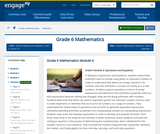Conditions of Use:
Remix and Share
Rating

In Module 4, Expressions and Equations, students extend their arithmetic work to include using letters to represent numbers in order to understand that letters are simply "stand-ins" for numbers and that arithmetic is carried out exactly as it is with numbers. Students explore operations in terms of verbal expressions and determine that arithmetic properties hold true with expressions because nothing has changedthey are still doing arithmetic with numbers. Students determine that letters are used to represent specific but unknown numbers and are used to make statements or identities that are true for all numbers or a range of numbers. They understand the relationships of operations and use them to generate equivalent expressions, ultimately extending arithmetic properties from manipulating numbers to manipulating expressions. Students read, write and evaluate expressions in order to develop and evaluate formulas. From there, they move to the study of true and false number sentences, where students conclude that solving an equation is the process of determining the number(s) that, when substituted for the variable, result in a true sentence. They conclude the module using arithmetic properties, identities, bar models, and finally algebra to solve one-step, two-step, and multi-step equations.

Subject:
Numbers and Operations
Material Type:
Module
Provider:
New York State Education Department
Provider Set:
EngageNY
12/17/2013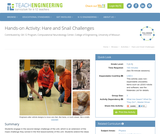Conditions of Use:
Rating

Students engage in the second design challenge of the unit, which is an extension of the maze challenge they solved in the first lesson/activity of this unit. Students extend the ideas learned in the maze challenge with a focus more on the robot design. Gears are a very important part of any machine, particularly when it has a power source such as engine or motor. Specifically, students learn how to design the gear train from the LEGO MINDSTORMS(TM) NXT servomotor to the wheel to make the LEGO taskbot go faster or slower. A PowerPoint® presentation, pre/post quizzes and a worksheet are provided.

Subject:
Engineering
Electronic Technology
Material Type:
Activity/Lab
Provider:
TeachEngineering
Provider Set:
TeachEngineering
Author:
Pranit Samarth
Sachin Nair
Satish S. Nair
09/18/2014Rating

This lesson unit is intended to help you assess how well students are able to: Perform arithmetic operations, including those involving whole-number exponents, recognizing and applying the conventional order of operations; Write and evaluate numerical expressions from diagrammatic representations and be able to identify equivalent expressions; apply the distributive and commutative properties appropriately; and use the method for finding areas of compound rectangles.

Subject:
Geometry
Material Type:
Assessment
Lesson Plan
Provider:
Shell Center for Mathematical Education
U.C. Berkeley
Provider Set:
Mathematics Assessment Project (MAP)
04/26/2013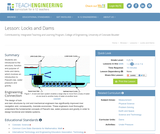Conditions of Use:
Rating

Students are introduced to the structure, function and purpose of locks and dams, which involves an introduction to Pascal's law, water pressure and gravity.

Subject:
Engineering
Hydrology
Material Type:
Lesson Plan
Provider:
TeachEngineering
Provider Set:
TeachEngineering
Author:
Denali Lander
Denise W. Carlson
Jeff Lyng
Kristin Field
Lauren Cooper
09/18/2014Conditions of Use:
Remix and Share
Rating

Subject:
Mathematics
Material Type:
Full Course
Provider:
Pearson
10/06/2016Conditions of Use:
Remix and Share
Rating

Expressions

Type of Unit: Concept

Prior Knowledge

Students should be able to:

Write and evaluate simple expressions that record calculations with numbers.
Use parentheses, brackets, or braces in numerical expressions and evaluate expressions with these symbols.
Interpret numerical expressions without evaluating them.

Lesson Flow

Students learn to write and evaluate numerical expressions involving the four basic arithmetic operations and whole-number exponents. In specific contexts, they create and interpret numerical expressions and evaluate them. Then students move on to algebraic expressions, in which letters stand for numbers. In specific contexts, students simplify algebraic expressions and evaluate them for given values of the variables. Students learn about and use the vocabulary of algebraic expressions. Then they identify equivalent expressions and apply properties of operations, such as the distributive property, to generate equivalent expressions. Finally, students use geometric models to explore greatest common factors and least common multiples.

Subject:
Mathematics
Algebra
Material Type:
Unit of Study
Provider:
Pearson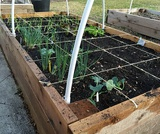Conditions of Use:
Remix and Share
Rating

Students use a rectangular area model to understand the distributive property. They watch a video to find how to express the area of a rectangle in two different ways. Then they find the area of rectangular garden plots in two ways.Key ConceptsThe distributive property can be used to rewrite an expression as an equivalent expression that is easier to work with. The distributive property states that multiplication distributes over addition.Applying multiplication to quantities that have been combined by addition: a(b + c)Applying multiplication to each quantity individually, and then adding the products together: ab + acThe distributive property can be represented with a geometric model. The area of this rectangle can be found in two ways: a(b + c) or ab + ac. The equality of these two expressions, a(b + c) = ab + ac, is the distributive property.Goals and Learning ObjectivesUse a geometric model to understand the distributive property.Write equivalent expressions using the distributive property.

Subject:
Algebra
Geometry
Material Type:
Lesson Plan
Provider:
Pearson
09/21/2015Conditions of Use:
Remix and Share
Rating

Students do a card sort in which they match expressions in words with their equivalent algebraic expressions.Key ConceptsA mathematical expression that uses letters to represent numbers is an algebraic expression.A letter used in place of a number in an expression is called a variable.An algebraic expression combines both numbers and letters using the arithmetic operations of addition (+), subtraction (–), multiplication (·), and division (÷) to express a quantity.Words can be used to describe algebraic expressions.There are conventions for writing algebraic expressions:The product of a number and a variable lists the number first with no multiplication sign. For example, the product of 5 and n is written as 5n, not n5.The product of a number and a factor in parentheses lists the number first with no multiplication sign. For example, write 5(x + 3), not (x + 3)5.For the product of 1 and a variable, either write the multiplication sign or do not write the "1." For example, the product of 1 and z is written either 1 ⋅ z or z, not 1z.Goals and Learning ObjectivesTranslate between expressions in words and expressions in symbols.

Subject:
Algebra
Material Type:
Lesson Plan
Provider:
Pearson
09/21/2015Conditions of Use:
Remix and Share
Rating

Students play an Expressions Game in which they describe expressions to their partners using the vocabulary of expressions: term, coefficient, exponent, constant, and variable. Their partners try to write the correct expressions based on the descriptions.Key ConceptsMathematical expressions have parts, and these parts have names. These names allow us to communicate with others in a precise way.A variable is a symbol (usually a letter) in an expression that can be replaced by a number.A term is a number, a variable, or a product of numbers and variables. Terms are separated by the operator symbols + (plus) and – (minus).A coefficient is a symbol (usually a number) that multiplies the variable in an algebraic expression.An exponent tells how many copies of a number or variable are multiplied together.A constant is a number. In an expression, it can be a constant term or a constant coefficient. In the expression 2x + 3, 2 is a constant coefficient and 3 is a constant term.Goals and Learning ObjectivesIdentify parts of an expression using appropriate mathematical vocabulary.Write expressions that fit specific descriptions (for example, the expression is the sum of two terms each with a different variable).

Subject:
Algebra
Material Type:
Lesson Plan
Provider:
Pearson
09/21/2015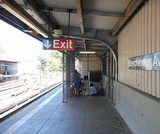Conditions of Use:
Remix and Share
Rating

Students critique the work of other students and revise their own work based on feedback from the teacher and peers.Key ConceptsConcepts from previous lessons are integrated into this unit task: rewriting expressions, using parentheses, and using the distributive property. Students apply their knowledge, review their work, and make revisions based on feedback from you and their peers. This process creates a deeper understanding of the concepts.Goals and Learning ObjectivesApply knowledge of expressions to correct the work of other students.Track and review the choice of strategy when problem solving.

Subject:
Mathematics
Material Type:
Lesson Plan
Provider:
Pearson
09/21/2015Conditions of Use:
Remix and Share
Rating

Students express the lengths of trains as algebraic expressions and then substitute numbers for letters to find the actual lengths of the trains.Key ConceptsAn algebraic expression can be written to represent a problem situation. More than one algebraic expression may represent the same problem situation. These algebraic expressions have the same value and are equivalent.To evaluate an algebraic expression, a specific value for each variable is substituted in the expression, and then all the calculations are completed using the order of operations to get a single value.Goals and Learning ObjectivesEvaluate expressions for the given values of the variables.

Subject:
Algebra
Material Type:
Lesson Plan
Provider:
Pearson
09/21/2015Conditions of Use:
Remix and Share
Rating

Students write an expression for the length of a train, using variables to represent the lengths of the different types of cars.Key ConceptsA numerical expression consists of a number or numbers connected by the arithmetic operations of addition, subtraction, multiplication, division, and exponentiation.An algebraic expression uses letters to represent numbers.An algebraic expression can be written to represent a problem situation. Sometimes more than one algebraic expression may represent the same problem situation. These algebraic expressions have the same value and are equivalent.The properties of operations can be used to make long algebraic expressions shorter:The commutative property of addition states that changing the order of the addends does not change the end result:a + b = b + a.The associative property of addition states that changing the grouping of the addends does not change the end result:(a + b) + c = a + (b + c).The distributive property of multiplication over addition states that multiplying a sum by a number gives the same result as multiplying each addend by the number and then adding the products together:a(b + c) = ab + ac.Goals and Learning ObjectivesWrite algebraic expressions that describe lengths of freight trains.Use properties of operations to shorten those expressions.

Subject:
Algebra
Material Type:
Lesson Plan
Provider:
Pearson
09/21/2015Conditions of Use:
Remix and Share
Rating

Students represent problem situations using expressions and then evaluate the expressions for the given values of the variables.Key ConceptsAn algebraic expression can be written to represent a problem situation.To evaluate an algebraic expression, a specific value for each variable is substituted in the expression, and then all the calculations are completed using the order of operations to get a single value.Goals and Learning ObjectivesDevelop fluency in writing expressions to represent situations and in evaluating the expressions for given values.

Subject:
Algebra
Material Type:
Lesson Plan
Provider:
Pearson
09/21/2015Conditions of Use:
Remix and Share
Rating

Getting Started

Type of Unit: Introduction

Prior Knowledge

Students should be able to:

Solve and write numerical equations for whole number addition, subtraction, multiplication, and division problems.
Use parentheses to evaluate numerical expressions.
Identify and use the properties of operations.

Lesson Flow

In this unit, students are introduced to the rituals and routines that build a successful classroom math community and they are introduced to the basic features of the digital course that they will use throughout the year.

An introductory card sort activity matches students with their partner for the week. Then over the course of the week, students learn about the lesson routines: Opening, Work Time, Ways of Thinking, Apply the Learning, Summary of the Math, and Reflection. Students learn how to present their work to the class, the importance of taking responsibility for their own learning, and how to effectively participate in the classroom math community.

Students then work on Gallery problems to further explore the program’s technology resources and tools and learn how to organize their work.

The mathematical work of the unit focuses on numerical expressions, including card sort activities in which students identify equivalent expressions and match an expression card to a word card that describes its meaning. Students use the properties of operations to identify equivalent expressions and to find unknown values in equations.

Subject:
Mathematics
Material Type:
Unit of Study
Provider:
Pearson